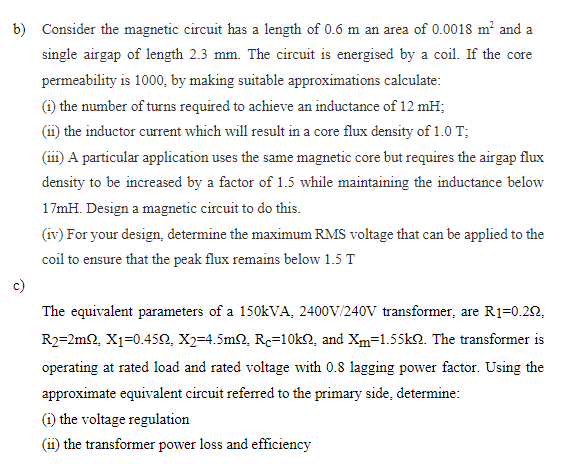# Question Solved1 Answer(b) (i) N = 110 turns; (ii) IL = 16.5 A (c) (i) regulation = 2.24% (ii) efficiency = 98.25% b) Consider the magnetic circuit has a length of 0.6 m an area of 0.0018 m? and a single airgap of length 2.3 mm. The circuit is energised by a coil. If the core permeability is 1000, by making suitable approximations calculate: (1) the number of turns required to achieve an inductance of 12 mH; (11) the inductor current which will result in a core flux density of 1.0 T; (111A particular application uses the same magnetic core but requires the airgap flux density to be increased by a factor of 1.5 while maintaining the inductance below 17mH. Design a magnetic circuit to do this. (iv) For your design, determine the maximum RMS voltage that can be applied to the coil to ensure that the peak flux remains below 1.5 T c) The equivalent parameters of a 150kVA, 2400V/240V transformer, are R1=0.20, R2=2m2, X1=0.4512, X2=4.5m... Re=10k12, and Xm=1.55k12. The transformer is operating at rated load and rated voltage with 0.8 lagging power factor. Using the approximate equivalent circuit referred to the primary side, determine: (1) the voltage regulation (11) the transformer power loss and efficiency(b) (i) N = 110 turns; (ii) IL = 16.5 A
(c) (i) regulation = 2.24% (ii) efficiency = 98.25%

Transcribed Image Text: b) Consider the magnetic circuit has a length of 0.6 m an area of 0.0018 m? and a single airgap of length 2.3 mm. The circuit is energised by a coil. If the core permeability is 1000, by making suitable approximations calculate: (1) the number of turns required to achieve an inductance of 12 mH; (11) the inductor current which will result in a core flux density of 1.0 T; (111A particular application uses the same magnetic core but requires the airgap flux density to be increased by a factor of 1.5 while maintaining the inductance below 17mH. Design a magnetic circuit to do this. (iv) For your design, determine the maximum RMS voltage that can be applied to the coil to ensure that the peak flux remains below 1.5 T c) The equivalent parameters of a 150kVA, 2400V/240V transformer, are R1=0.20, R2=2m2, X1=0.4512, X2=4.5m... Re=10k12, and Xm=1.55k12. The transformer is operating at rated load and rated voltage with 0.8 lagging power factor. Using the approximate equivalent circuit referred to the primary side, determine: (1) the voltage regulation (11) the transformer power loss and efficiency
More
Transcribed Image Text: b) Consider the magnetic circuit has a length of 0.6 m an area of 0.0018 m? and a single airgap of length 2.3 mm. The circuit is energised by a coil. If the core permeability is 1000, by making suitable approximations calculate: (1) the number of turns required to achieve an inductance of 12 mH; (11) the inductor current which will result in a core flux density of 1.0 T; (111A particular application uses the same magnetic core but requires the airgap flux density to be increased by a factor of 1.5 while maintaining the inductance below 17mH. Design a magnetic circuit to do this. (iv) For your design, determine the maximum RMS voltage that can be applied to the coil to ensure that the peak flux remains below 1.5 T c) The equivalent parameters of a 150kVA, 2400V/240V transformer, are R1=0.20, R2=2m2, X1=0.4512, X2=4.5m... Re=10k12, and Xm=1.55k12. The transformer is operating at rated load and rated voltage with 0.8 lagging power factor. Using the approximate equivalent circuit referred to the primary side, determine: (1) the voltage regulation (11) the transformer power loss and efficiency